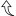This course enables students to consolidate, and continue to develop, an understanding of mathematical concepts related to number sense and operations, algebra, measurement, geometry, data, probability and financial literacy. Students will use mathematical processes, mathematical modelling, and coding to make sense of the mathematics they are learning and to apply their understanding to culturally responsive and relevant real-world situations. Students will continue to enhance their mathematical reasoning skills, including proportional reasoning, spatial reasoning, and algebraic reasoning, as they solve problems and communicate their thinking.

Credit Value 1.0, Prerequisite: None

## Opening Week ActivitiesLesson Hints Solution Check Your Understanding Video Bridge Problem Hint 1, Hint 2, Hint 3 Solution From Ted Ed: https://www.youtube.com/watch?v=7yDmGnA8Hw0 Gold Bar Problem

## Story-TellingLesson Video Activities Check Your Understanding Other Resources Wheat on a Chessboard (Exponents, Exponental Growth) Circumference of Earth (Parallel Lines, Circle formulas)

# Coding in the ClassrooM

### Google SheetsLesson Video Activities Homework Questions An old version from Excel Fill Down, *, +, -, \ Multiplication Table Fill Down (Tip Calculator) Multiplication Table Geometry Formulas Fill Down Practice Student Work Average, Sum, Min, Max Formula Intro Unit 6 Data, 2A, 2B Student Work Box and Whisker, Quartiles Candlestick graph Unit 6 Data, 2C, 2D Scatterplots, y=mx+b Slope, Yint, Line of Best Fit Unit 6 Data, 3A, 3D Video, Student Work, Another, Still another Correlation Coefficient (r & r^2) r, r^2, adding to graph Area Worksheet Own functions + Variables Assignment, Student Work, Adding a Dialog Box Vlookup Video 1, Video 2, Student Work Histograms Video, Student Work, Review Compounded Interest Mortgage Calculator, Assignment Law of Large Numbers Student Work, Assignment Pivot Table Student Work, Assignment And, Or, Not Student Work Ifs Student Work SumIf, CountIf Student Work Venn Diagrams Student Work, Review Pie Charts Review Pictographs Assignment, Lesson

### SpherosLesson Video Activities Check Your Understanding Other Resources Introduction Intro Grid Intro Form Loops Loops Grid Math, Variables Math Variable Grid Variables Form Math Form Shapes Shapes Grid Inequalities, Ifs

# Units of Study

## Integer, Number Sense and OperationsLesson Curriculum Activities Check Your Understanding Questions Other Resources

## AlgebraLesson Curriculum Activities Check Your Understanding Questions Other Resources

## EquationsLesson Curriculum Activities Check Your Understanding Questions Other Resources

### Linear and Non-LinearLesson Curriculum Activities Check Your Understanding Questions Other Resources

## Analytic GeometryLesson Curriculum Activities Check Your Understanding Questions Other Resources